Code

Code

## C

$$dis((a,b),(c,d)) = \begin{cases} \max\{|a-c|,|b-d|\},& (a-c)(b-d) \geq 0\\ |a-c|+|b-d|,& (a-c)(b-d) < 0 \end{cases}$$

$$\forall i \in [1,n],\max\{|x-x_i|,|y-y_i|\} \leq mid$$

$$|x-x_i|+|y-y_i| \leq mid$$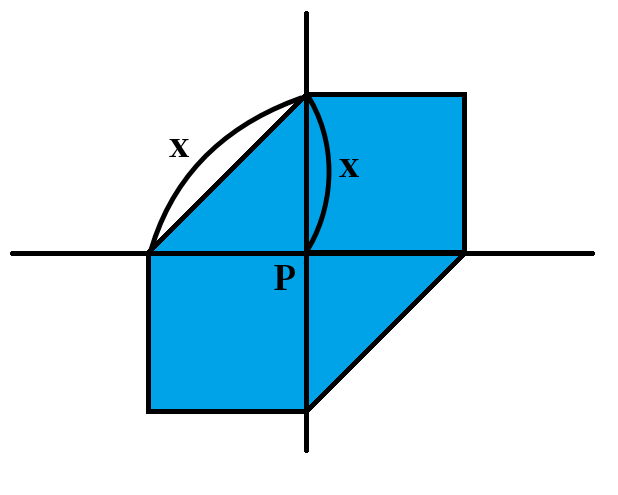（这里的 $x$ 就是二分的答案 $mid$）

\begin{aligned} &|x-x_i| \leq mid,|y-y_i| \leq mid\\ &\Rightarrow -mid \leq x-x_i \leq mid,-mid \leq y-y_i \leq mid\\ &\Rightarrow x \in [-mid+x_i,mid+x_i],y \in [-mid+y_i,mid+y_i] \end{aligned}

\begin{aligned} &|x-x_i-y+y_i| \leq mid\\ &\Rightarrow -mid \leq x-x_i-y+y_i \leq mid\\ &\Rightarrow -mid+x_i-y_i \leq x-y \leq mid+x_i-y_i \end{aligned}

Code

Code

## E

### 感性理解题目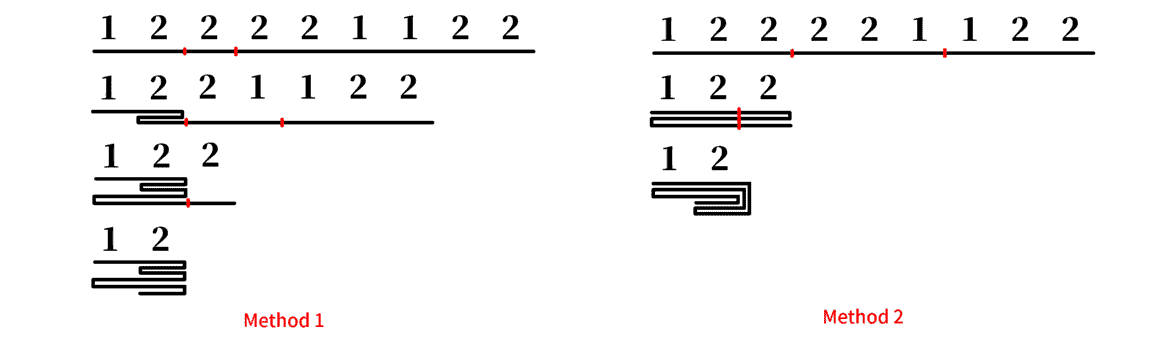### X-Y-X 串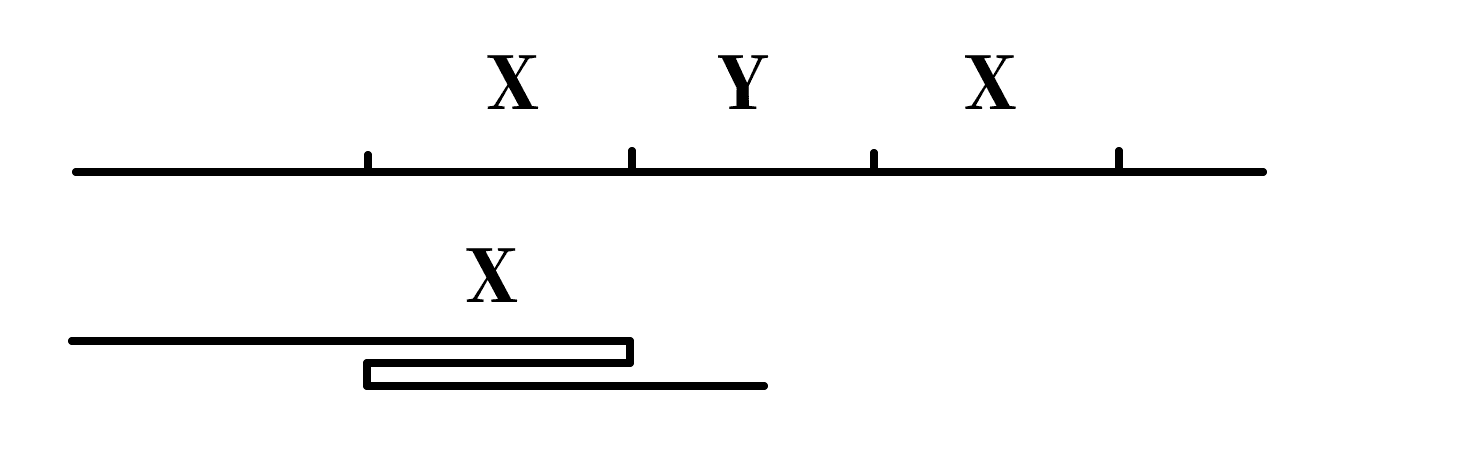1. 如何快速找出 X-Y-X 型区间？
2. 如何处理没有 X-Y-X 型区间的串？

#### 如何找出所有 X-Y-X 串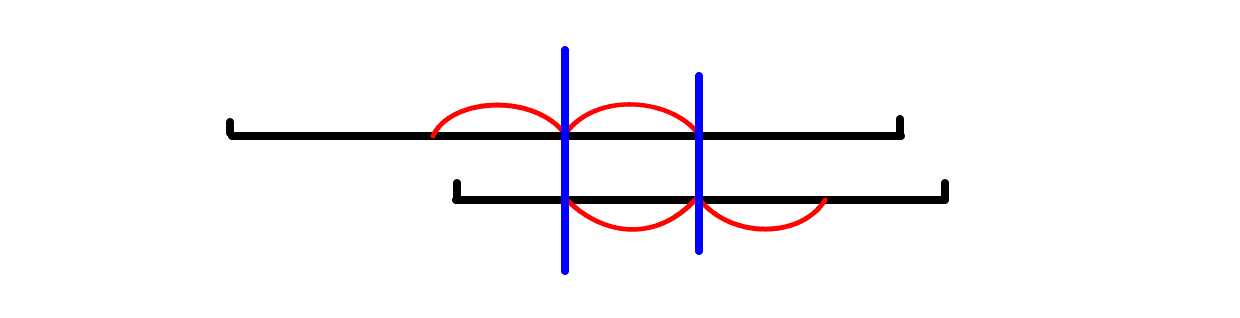1. 黑和红：把红对称到黑色的 $Y$ 里，$b$ 内有 X-Y-X 串，矛盾。
2. 黑和蓝：蓝的 $XY$ 和黑的 $YX$ 满足引理 $1$，矛盾。
3. 黑和绿：绿的 $XY$ 和黑的 $XY$ 满足引理 $1$，矛盾。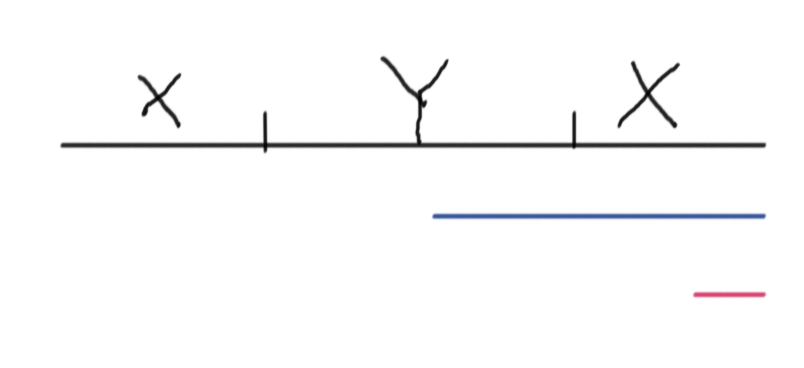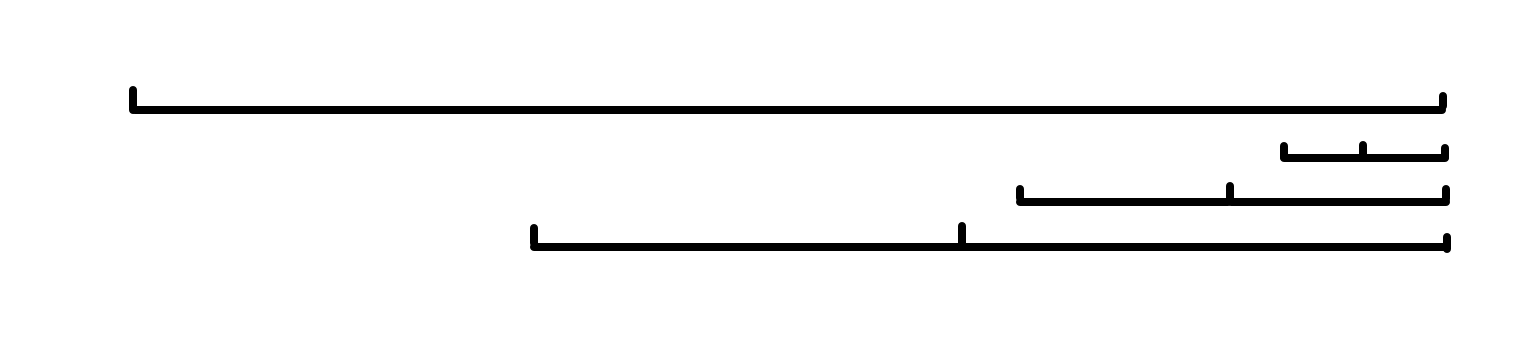• 如果出现了新的 X-Y-X 型的串：记录下来，并且将对应的后缀删去
• 如果未出现 X-Y-X 型串：就去更新下面即将要说的其他信息就好了。

#### 如何处理没有 X-Y-X 型区间的串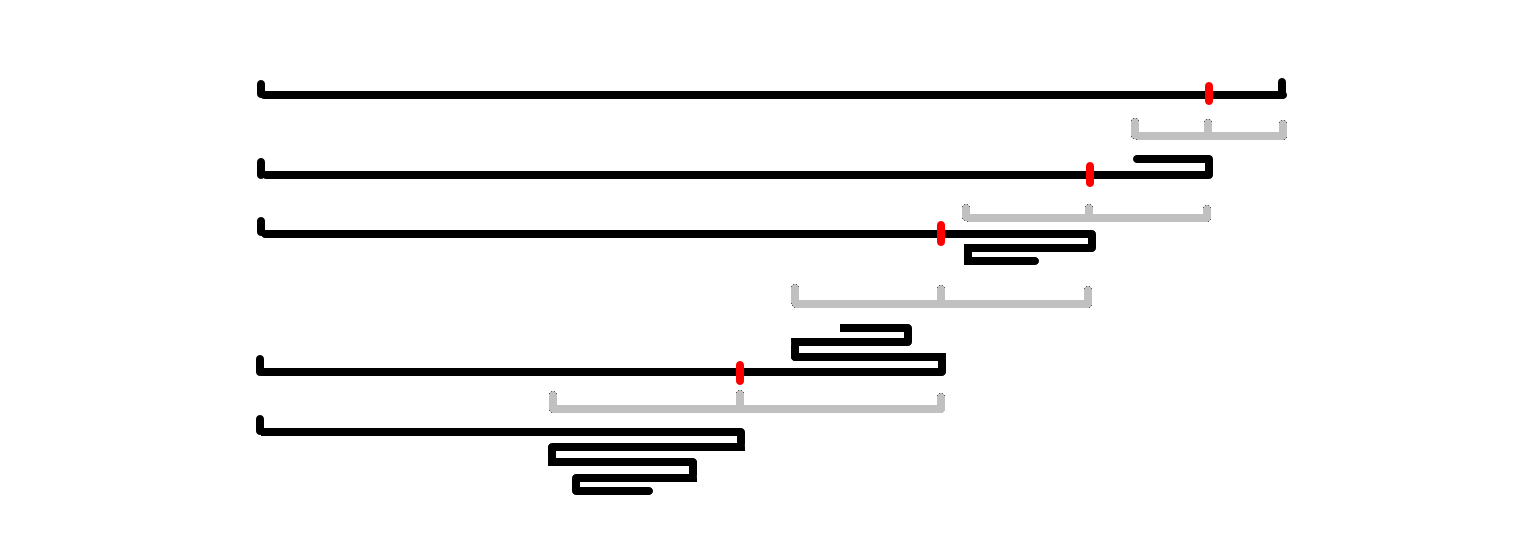• 对于后缀：从 vector 中就可以得到最短偶回文后缀。
• 对于前缀：设 $q_i$ 表示区间 $[1,i]$ 从开头折叠能最远折到哪里，$c_i$ 表示折叠次数。

• 对于前缀：如果可以继续跳的话一定代表了 $[q_i,now]$ 是一个偶回文串，直接判断即可
• 对于后缀：直接用 vector 信息即可，不需要特别维护。

### 完成了！

• 如果产生了新的 X-Y-X 串（一定是一个后缀）：直接删除掉一些后缀即可。
• 如果未产生新的 X-Y-X 串：更新 $q_i,c_i$ 即可。

Code

Last modification：August 27th, 2020 at 08:39 am